01 Sept 2023

Posted on:

24 Aug 2023

0

# Resolved:Rejecting Null Hypothesis Issue in Customer Engagement Project Task 4.

Hello, I hope you're all doing well.

In project problem task 4, there's an issue highlighted with red circles. We have two decision rules to either accept or reject the null hypothesis:

1) If the T-score is less than or equal to the negative critical t-value, we reject the null hypothesis. Otherwise, we accept it.
2) If the p-value is less than or equal to 0.05, we reject the null hypothesis. Otherwise, we accept it.

For students on the paid plan, this verification is working correctly. However, for students on the free plan, there's a discrepancy. When applying the first decision rule, it suggests accepting the null hypothesis. However, considering the second decision rule, the p-value is nearly 0.000, indicating that we should reject the null hypothesis.

What should we do in such case. I'm attaching picture for reference.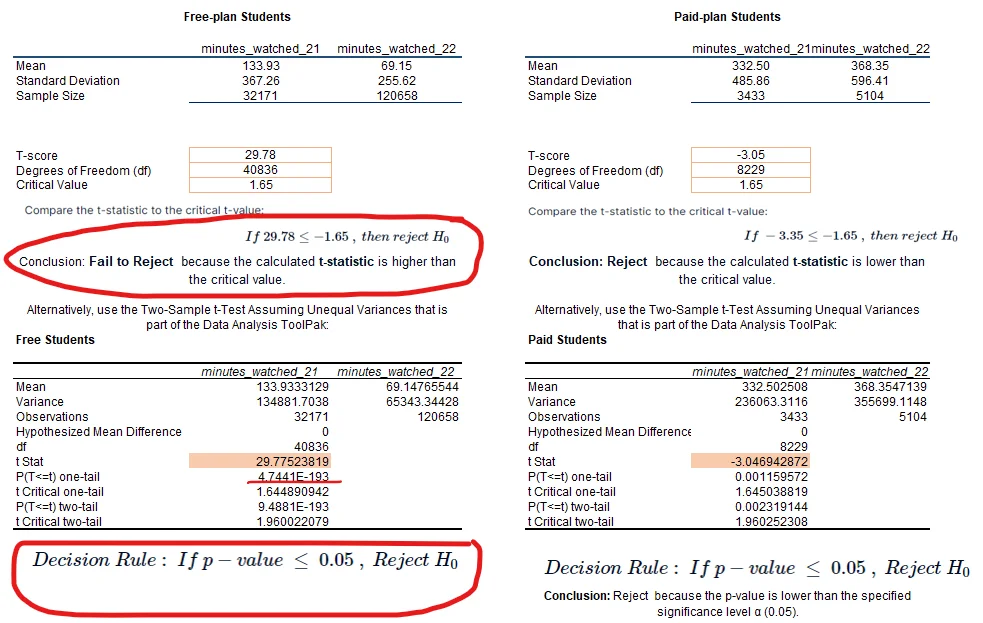Instructor
Posted on:

25 Aug 2023

0

Thank you for reaching out with your concerns.

I understand the confusion surrounding the T-score interpretation for the free-plan students you mentioned (as shown in the left-side picture). The T-score given is 29.78. As you rightly pointed out in the decision rule, a left-tailed test should be conducted. This means the T-score should be compared to the negative critical value. However, it appears that Excel is performing a right-tailed test, comparing the T-score with the positive critical value. This discrepancy explains why the p-value appears so low.

When interpreting these results, it's crucial to exercise discretion. It's worth noting that with experience, such nuances become more apparent, and you'll be better equipped to spot them.

I hope this clarification assists you in your understanding.

Warm regards,

The 365 Team

Posted on:

27 Aug 2023

0These pictures are of the solutions as provided when we submit the project.

I didn't get it. As I mentioned before.

Even if we use online calculator as mentioned in the lectures to find p value, we get .00001 (p-value)  it is still less than 0.05 so we reject the null by rule 2. But rule 1 is saying failed to reject null. So what should we do in such cases.

We have two decision rules to either accept or reject the null hypothesis:

1) If the T-score is less than or equal to the negative critical t-value, we reject the null hypothesis. Otherwise, we accept it.
2) If the p-value is less than or equal to 0.05, we reject the null hypothesis.

Instructor
Posted on:

28 Aug 2023

0

Thanks for reaching out!

You correctly outline the two  rules:
1) If the T-score is less than or equal to the negative critical t-value, we reject the null hypothesis. Otherwise, we accept it.
2) If the p-value is less than or equal to 0.05 (or any other significance level), we reject the null hypothesis.

In general, the two rules should coincide. Put simply, there should not be any difference for the outcome.

Let me alaborate further on the case that you mentioned.

Your null hypotheses should include the following:

·        The engagement (minutes watched) in Q4 2021 is higher than or equal to the one in Q4 2022 (μ1 ≥ μ2). We test free-plan and paying students separately.

Put simply the alternative hypothesis should be that the average minutes watched in Q4 2021 should be lower than the average minutes watched in Q4 2022.

What this means is that you should perform left-tailed one sided test. Therefore, you should compare the t-statistic to negative critical value. If it's less than that, we reject the null hypothesis. For reference use this calculator to perform the test.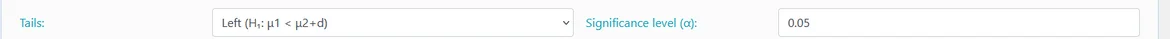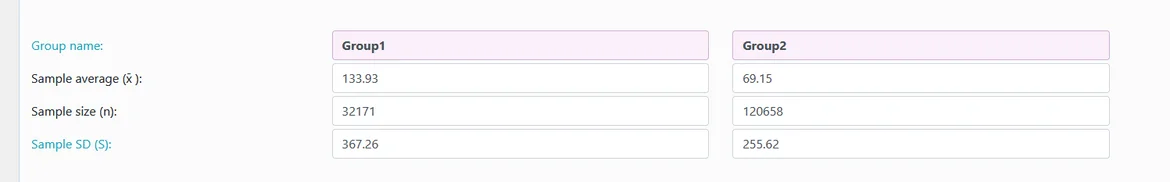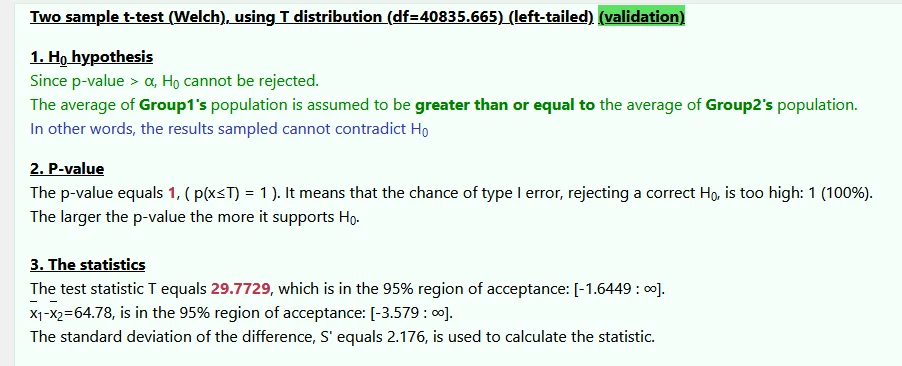As you can see the p value is above 0.05, so you cannot reject the hypothesis.

In the coming days we will also launch our version of the calculator that you can use but the alternative calculator should do the purpose for now.

Hope this helps!

Best,

The 365 Team

Instructor
Posted on:

28 Aug 2023

1

Here is some more information on the difference between two-sided and one sided tests that you can find useful:

Тhere are two types of hypotheses: two-sided and one-sided hypotheses.

A two-sided hypothesis, also known as a two-tailed, determines if there is a significant difference between the means of two groups without specifying the direction. The null hypothesis assumes that the means are equal, while the alternative hypothesis states that they’re not.

On the other hand, we use a one-sided (or one-tailed) hypothesis to calculate whether one mean is greater than or less than the other. In fact, the null hypothesis states that one mean is less than or equal to the other mean, while the alternative hypothesis states that one mean is greater than the other mean.

Please also note that we have developed multiple calculators that you can you as part of your studies on statistics. You can find them here.

Hope this helps.

Best,

The 365 Team

Posted on:

01 Sept 2023

0

Hi Ivan,

Thank you for your feedback. Your message helped me understand the issue better.

It's clear that there are some important details missing in the Excel solution, which led to the problem being raised in the first place. Additionally, the discrepancy in the p-value calculations between the suggested calculator in the lectures and the Excel solution is something worth noting. I assume the suggested calculator was performing a right-tailed test as well, which explains the difference in the p-values.

Please suggest another calculator that performs proper calculations. Like you used to perform the calculations.

Lastly, I suggest to include a p-value calculator for the t-score value as well in 365data science calculator menu, in addition to the existing one for the z-score. This enhancement would make the tool even more useful.

Thank you again for your help.

Instructor
Posted on:

01 Sept 2023

0

Thanks for the suggestions!

Please check the p-value calculator that we have developed. It includes all the four distributions that you will ever need.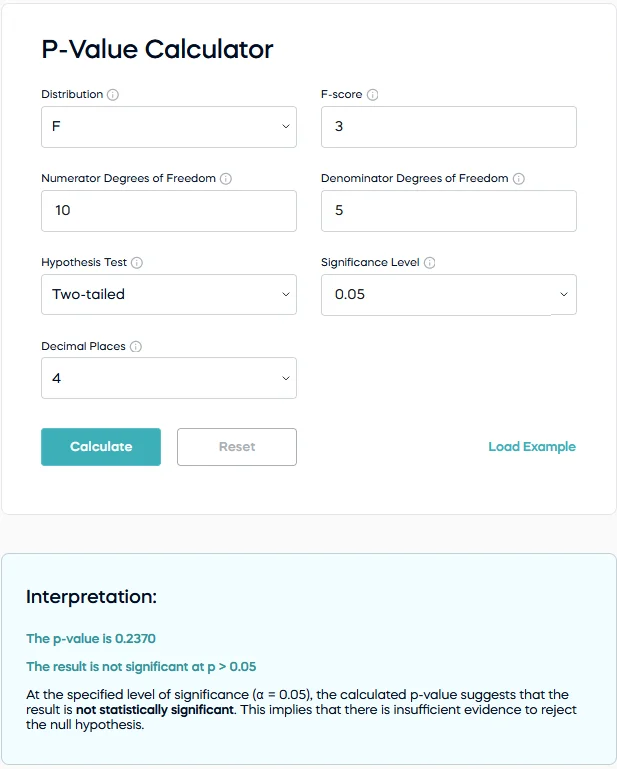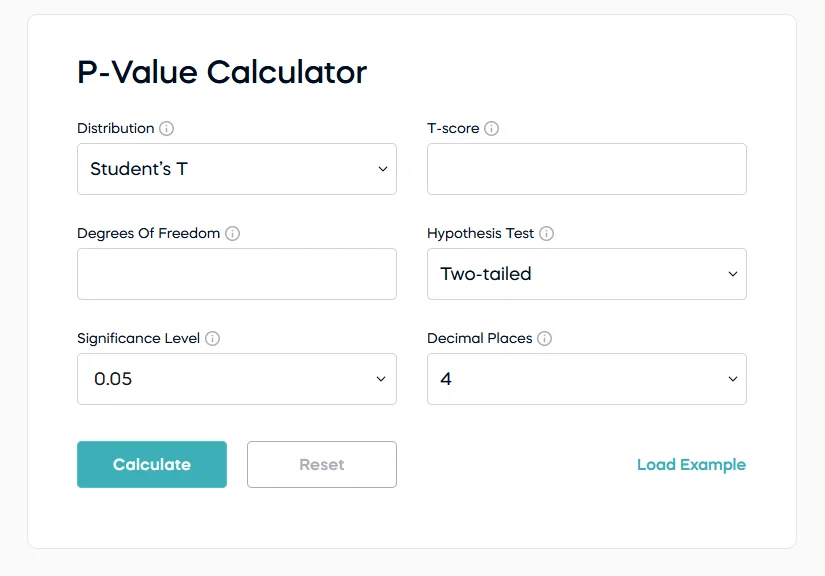You have to select from the dropdown the distrution and then type in the score. I suggets that you try it. I would be grateful for your feedback on the calculator as well!

Best,

The 365 Team